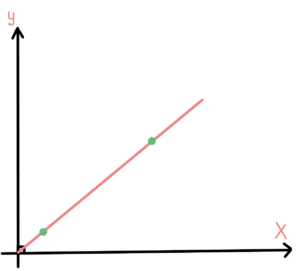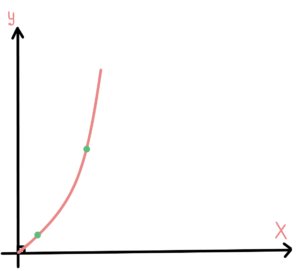# C# Time Complexity### Intro

In this article, you will learn how to calculate C# time complexity to measure the overall performance of your loops, recursive functions, and other algorithms. By understanding the time complexity of a given algorithm, developers can make informed decisions about which algorithms to use in their code and can optimize their code to improve performance. This article will explore key concepts and techniques for calculating time complexity in C#.

### Big “O”

First, it is important to understand the basic concepts of time complexity. Time complexity is a measure of the amount of time an algorithm takes to complete, as a function of the size of the input. The most common way to express time complexity is using big O notation, which describes the upper bound on the time complexity of an algorithm. For example, a time complexity of O(n) means that the algorithm takes at most n steps to complete, where n is the size of the input.

FLASH SALES
\$29.95
NEW
\$249.00
Become a C# PRO and land your first developer job!

However, when calculating time complexity in C#, it is important to consider both the best-case and worst-case scenarios. The best-case scenario is the scenario in which the algorithm completes in the least amount of time. In contrast, the worst-case scenario is the scenario in which the algorithm takes the longest to complete. By considering both scenarios, developers can get a more accurate understanding of the overall performance of an algorithm.

### How to measure the time complexity of a loop?

One of the most important techniques for calculating time complexity in C# is analyzing the loops in an algorithm. Loops are a common source of inefficiencies in algorithms, and understanding the time complexity of loops is crucial for optimizing code. For example, a for loop that iterates through an array has a time complexity of O(n), where n is the size of the array.

An example of a simple for loop that iterates through an array:

In this example, the algorithm’s time complexity is O(n), where “n” is the size of the array. This is because the loop iterates through the entire array once, and the amount of time it takes to complete is directly proportional to the size of the array.

On the other hand, a nested for loop that iterates through two arrays has a time complexity of O(n*m), where n is the size of the first array and m is the size of the second array.

On the picture below, you can see how the complexity grows with the size of input:An example of a nested for loop that iterates through two arrays:

In this example, the algorithm’s time complexity is O(n^2), where “n” is the size of the array. This is because the outer loop iterates through the array, and the inner loop iterates through the same array, effectively multiplying the size of the input.

And here is the graph:### How to measure the time complexity of a recursive function?

Another technique for calculating time complexity in C# is analyzing the recursive calls in an algorithm. Recursive algorithms are often used to solve complex problems, but they can also be a source of inefficiencies. To calculate the time complexity of a recursive algorithm, developers can use the master theorem, which is a mathematical formula that can be used to calculate the time complexity of recursive algorithms.

An example of a simple recursive function that calculates the factorial of a number:

In this example, the time complexity of the algorithm can be calculated using the master theorem as T(n) = T(n-1) + O(1), which results in O(n). As the function calls itself with a reduced value of n in each recursion, the amount of work done increases linearly with the size of the input.

### Are there any tools to measure the performance?

In addition to analyzing loops and recursive calls, developers can also use tools such as profilers to measure the actual performance of an algorithm in C#. Profilers are software tools that can measure the amount of time an algorithm takes to complete, as well as the amount of memory it uses. By using a profiler, developers can get a more accurate picture of the performance of their code and can make more informed decisions about optimizing it.

An example of using a profiler to measure the performance of an algorithm:

In this example, the `Stopwatch` class is used to measure the amount of time it takes for the algorithm (the for loop) to complete. By calling the `Start()` and `Stop()` methods, the time elapsed can be obtained from the `ElapsedMilliseconds` property and printed out.

### Conclusion

In conclusion, calculating time complexity in C# is an important aspect of software development that helps developers determine an algorithm’s efficiency. By understanding the concepts of time complexity, analyzing loops and recursive calls, and using tools such as profilers, developers can make informed decisions about which algorithms to use in their code and can optimize their code to improve performance.

You can learn even more following our Progress Academy